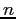Simple User Solutions to the C. S. Problem

Tom Kelliher, CS 311

Mar. 21, 2012

Announcements:

From last time:

1. Introduction to process synchronization.

Outline:

1. Software solutions for two processes.

2. Software solution forprocesses.

Assignment:

Software Solutions

Two Process Solutions

Assumptions:

1. Only two cooperating processes.

2. We have P0 and P1.

3. Replace i with appropriate integer.

Try 1

```int turn = 0;        // Shared control variable.

// mutexbegin:
while (turn != i)
;                 // Busy wait.

// mutexend:
turn = 1 - i;
```

1. Guarantees mutual exclusion.

2. Does not guarantee progress -- enforces strict alternation of processes entering CS's.

3. Bounded waiting violated -- suppose one process terminates while its its turn?

Try 2

Remove strict alternation requirement.

```int flag = { FALSE, FALSE }   // flag[i] indicates that Pi is in its
//  critical section.

// mutexbegin:
while (flag[1 - i])
;
flag[i] = TRUE;

// mutexend:
flag[i] = FALSE;
```

1. Mutual exclusion violated.

2. Progress ok.

3. Bounded waiting?

Try 3

Restore mutual exclusion.

```int flag = { FALSE, FALSE }   // flag[i] indicates that Pi wants to
//  enter its critical section.

// mutexbegin:
flag[i] = TRUE;
while (flag[1 - i])
;

// mutexend:
flag[i] = FALSE;
```

1. Guarantees mutual exclusion.

2. Violates progress -- both processes could set flag and then deadlock on the while.

3. Bounded waiting?

Try 4

```int flag = { FALSE, FALSE }   // flag[i] indicates that Pi wants to
//  enter its critical section.

// mutexbegin:
flag[i] = TRUE;
while (flag[1 - i])
{
flag[i] = FALSE;
delay;                  // Sleep for some time.
flag[i] = TRUE;
}

// mutexend:
flag[i] = FALSE;
```

1. Mutual exclusion guaranteed.

2. Progress violated (processes can ``dance'').

3. Bounded waiting violated.

Peterson's Algorithm

```int flag = { FALSE, FALSE }   // flag[i] indicates that Pi wants to
//  enter its critical section.
int turn = 0;                    // turn indicates which process has
//  priority in entering its critical
//  section.

// mutexbegin:
flag[i] = TRUE;
turn = 1 - i;
while (flag[1 - i] && turn == 1 - i)
;

// mutexend:
flag[i] = FALSE;
```

1. Satisfies all solution requirements. Why?

Multiple Process Solution

Lamport's Bakery algorithm.

Assumptions:

1. NPROCS is the number of processes.

2. max(int *array) returns the maximum value in array.

3. Each process has a unique ID, so ties on the number chosen are broken by comparing IDs.

4. Replace i with the appropriate process ID.

```// Global initialization:
int choosing[NPROCS] = { FALSE };
int number[NPROCS] = { 0 };

// mutexbegin:
choosing[i] = TRUE;
number[i] = max(number) + 1;
choosing[i] = FALSE;
for (j = 0; j < NPROCS; ++j)
{
while (choosing[j])
;

while (number[j] != 0 && (number[j] < number[i] ||
number[j] == number[i] && j < i) )
;
}

// mutexend:
number[i] = 0;
```

1. Is it correct?

2. What can happen to number? Is that likely?

Thomas P. Kelliher 2012-03-20
Tom Kelliher